# analog .pdf

### File information

Original filename: analog.pdf
Title: EXAMINATION PAPER
Author: Staff Use

This PDF 1.5 document has been generated by Microsoft® Office Word 2007, and has been sent on pdf-archive.com on 11/11/2010 at 11:22, from IP address 220.244.x.x. The current document download page has been viewed 1216 times.
File size: 197 KB (5 pages).
Privacy: public file

analog.pdf (PDF, 197 KB)

### Document preview

EXAMINATION
PAPER
ID NUMBER_______________
SEMESTER:

YEAR

2

FACULTY/SCHOOL:

SEAT NO:_________
2009

CAMPUS

SCHOOL

Health, Engineering and
Science
SUBJECT CODES

Engineering and Science

SUBJECT TITLES

VEF2004

(PLEASE LIST ALL CODES AND TITLES

C
F
M S
W B
WHERE EXAM WILL BE SAT

Systems and Applications 2D

FOR WHICH THIS PAPER IS TO BE USED)

DURATION OF EXAMINATION

3

THIS EXAMINATION COUNTS FOR

HOURS

60 %

OF THE ASSESSMENT IN THIS SUBJECT.

EXAMINATION PAPERS DETAILS
TOTAL NUMBER OF PAGES (including this one)

5

NUMBER OF QUESTIONS

8

NUMBER OF QUESTIONS TO BE ATTEMPTED

All

SPECIAL INSTRUCTIONS TO CANDIDATES

Use a separate answer booklet for each
section.
EXAMINATION MATERIAL PERMITTED TO BE USED BY CANDIDATES :
Section A: Analogue Electronics, Text Book “Microelectronic Circuits”, Sedra &amp; Smith.
Section B: Digital Electronics, Two A4 pages (sides) of written material.
Section C: Energy Conversion, Two A4 pages (sides) of written material.
EXAMINATION MATERIAL SUPPLIED BY STUDENT ADMINISTRATION
YES

NO



YES

NO



CAN THE EXAM PAPER BE RELEASED TO STUDENTS

YES

NO



CAN THE EXAM PAPER BE RELEASED TO LIBRARY

YES

NO



YES

3

NO

GRAPH PAPER

EXAMINER/S

A.Zayegh/Y.Ng/JShi

EXT

MODERATOR/S

C.Ozansoy/J.Chlond/
W.S.Lee

EXT

4858/4724
/4034
5046/4701/
4053

CAMPUS

Footscray

CAMPUS

Footscray

(Examiner/s must be available on this number for duration of this examination)

VEF2004 Systems and Applications 2D (Section A, B &amp; C)

November 2009
Page 1

IMPORTANT INSTRUCTIONS TO STUDENTS

SECTION A IS THE ANALOGUE ELECTRONCS SECTION

SECTION B IS THE DIGITAL ELECTRONICS SECTION
SECTION C IS THE ENERGY CONVERSION SECTION

SECTION A: Analogue Electronics
Question A1. (Frequency Response) (20 Marks)
An MOSFET common-source amplifier
has Rin = 2 MΩ, gm = 4 mA/V, ro = 100 kΩ,
RD = 10 kΩ, Cgs = 2 pF. And Cgd = 0.5 pF. The amplifier is fed from a voltage source with
internal resistance of 500 kΩ and is connected to a 10-kΩ load.
a) Draw the circuit and label all components
b) Find the overall mid-band gain AM
c) Find the upper 3-dB frequency fH.
d) If we want to double fH what is the most appropriate components to be change.

Question A2 (Differential Amplifier) (20 Marks)
Figure-1 is a BJT differential amplifier using transistors with  = 100 and VA = 100 V.
a) Find the differential gain and the differential input resistance
b) Find the common-mode gain

Figure-1

Question A3 (Amplifier stability) (20 Marks)
An op amp designed to have a low-frequency gain of 105 and high-frequency response
dominated by a single pole at ω = 100 rad/s, acquires, through a manufacturing error, a pair of
a) At what frequency does the total phase shift reach 180o?
b) At this frequency, for what value of β, assumed to be frequency independent?

SECTION B: Digital Electronics
Please see the separate pdf file for the Digital part of the exam.

SECTION C: Energy Conversion
Question 1 (10 marks)
Consider the magnetic structure shown in Figure 1. Find the current in the 50-turn winding to
set up a flux density of 0.8T in the structure, assuming that the relative permeability of the
core material is 2000. Use the concept of reluctance in your solution.

Figure 1: Magnetic structure for Question 1.
Question 2 (20+10=30 marks)
A 15kVA 2300V/230V transformer is to be tested to determine its excitation branch
components, its series impedances, and its voltage regulation. The following data have been
taken from the primary side of the transformer:
OPEN-CIRCUIT TEST
Voc=2300 V
Ioc=0.21A
Poc=50W

SHORT-CIRCUIT TEST
Vsc=47V
Isc=6.0A
Psc=160W

a) Find the equivalent circuit of this transformer referred to the high voltage side (primary)
and draw the equivalent circuit diagram;
(20 marks)
b) Determine the efficiency of the transformer at full load with a lagging power factor of 0.8.
(10 marks)
Question 3 (20 marks).
a) How many methods can be used for speed control of a DC motor?

(5 marks)

b) The armature resistance of a 220 V shunt motor is 2. At no load, the line current is 2.4 A
at rated voltage. The field current is 0.8 A, and the speed is 1440 rpm. Find the motor
speed when the line current increases to 12.8 A, the field current remaining the same.
Assume that armature reaction does not affect the field flux.
(10 marks)
c) The counter emf of a shunt motor is 227V, the field resistance is 160, and the field
current is 1.5A. The line current is 36.5 A. Find the armature resistance. If the line current
during starting must be limited to 60A, how much starter resistance must be added in
series with the armature?
(5 marks)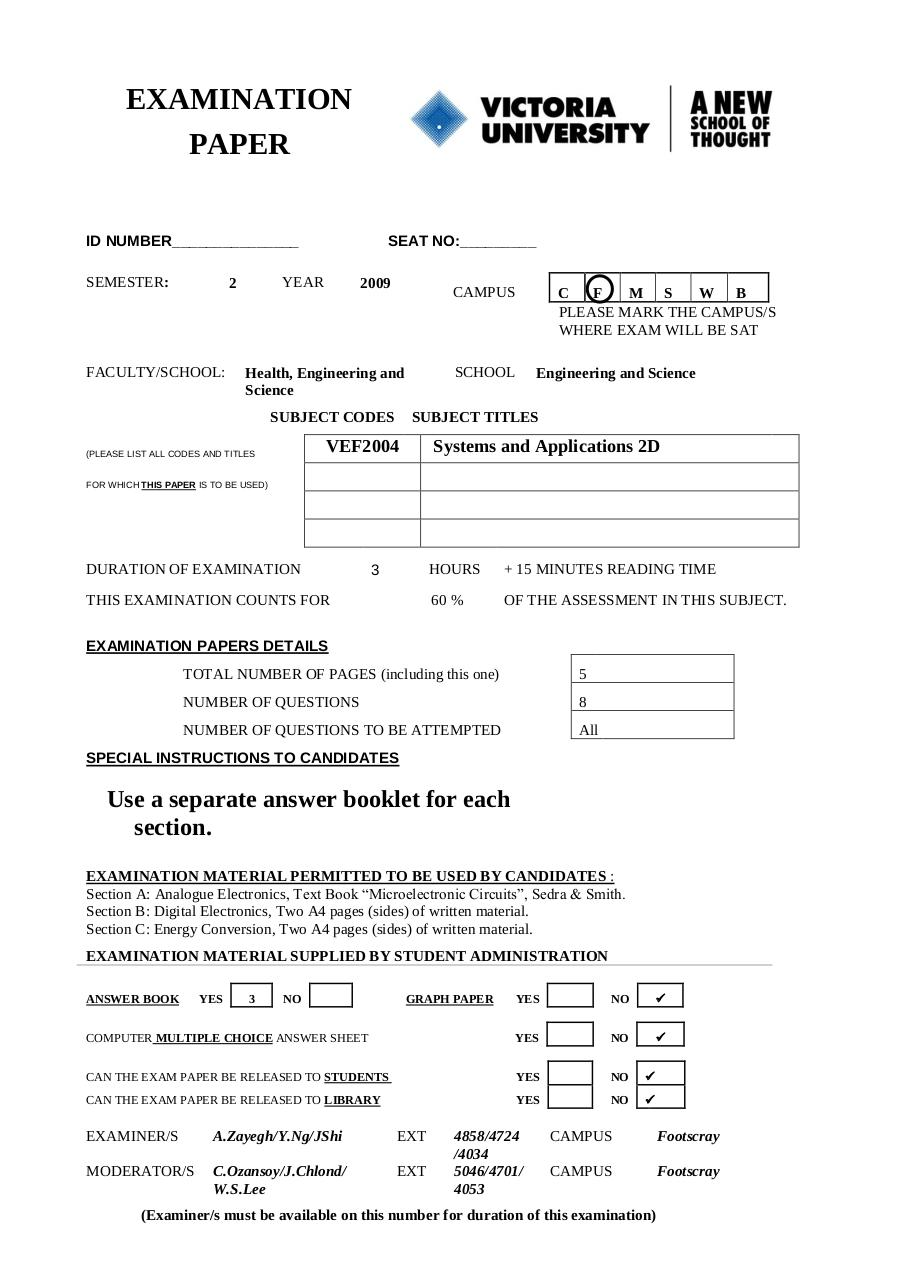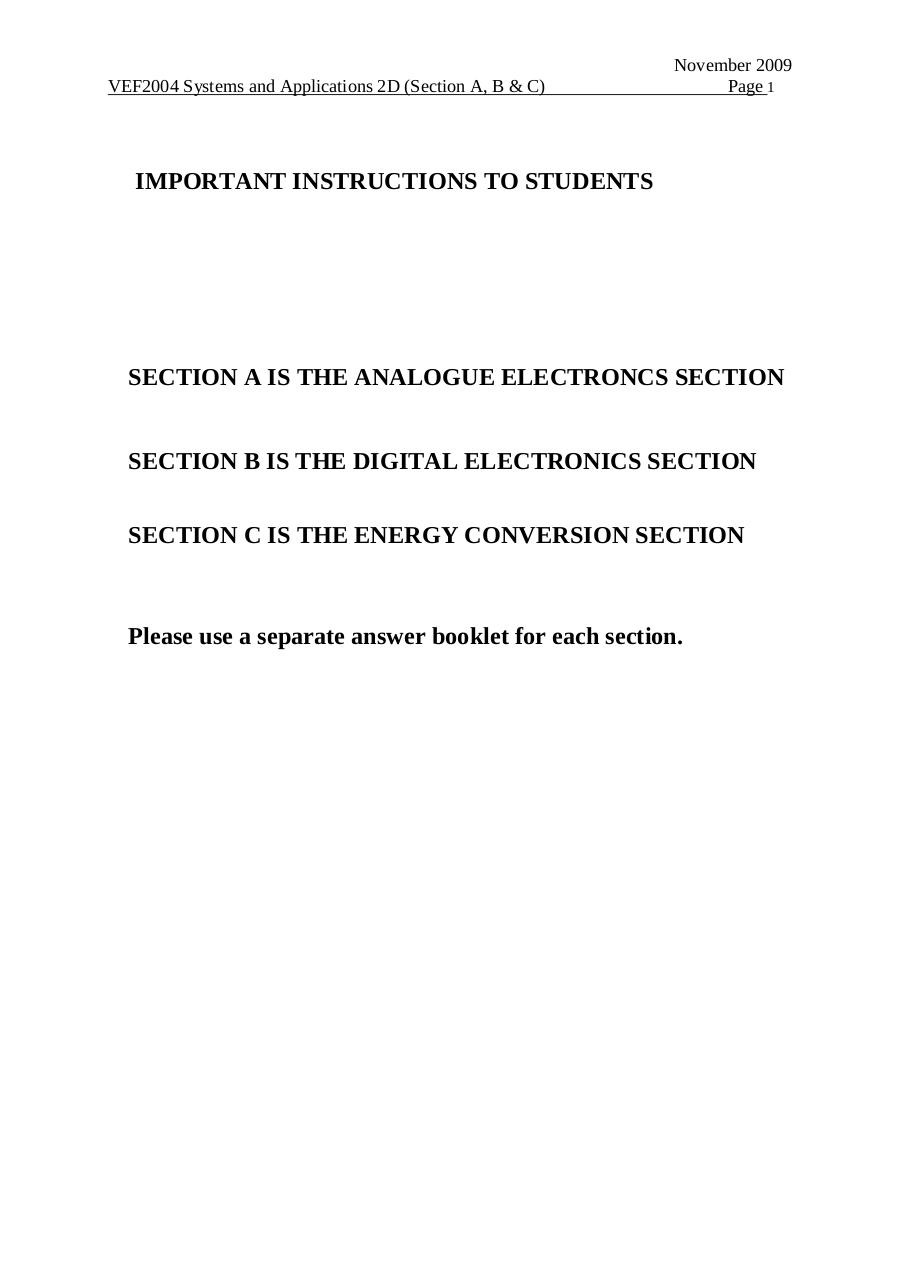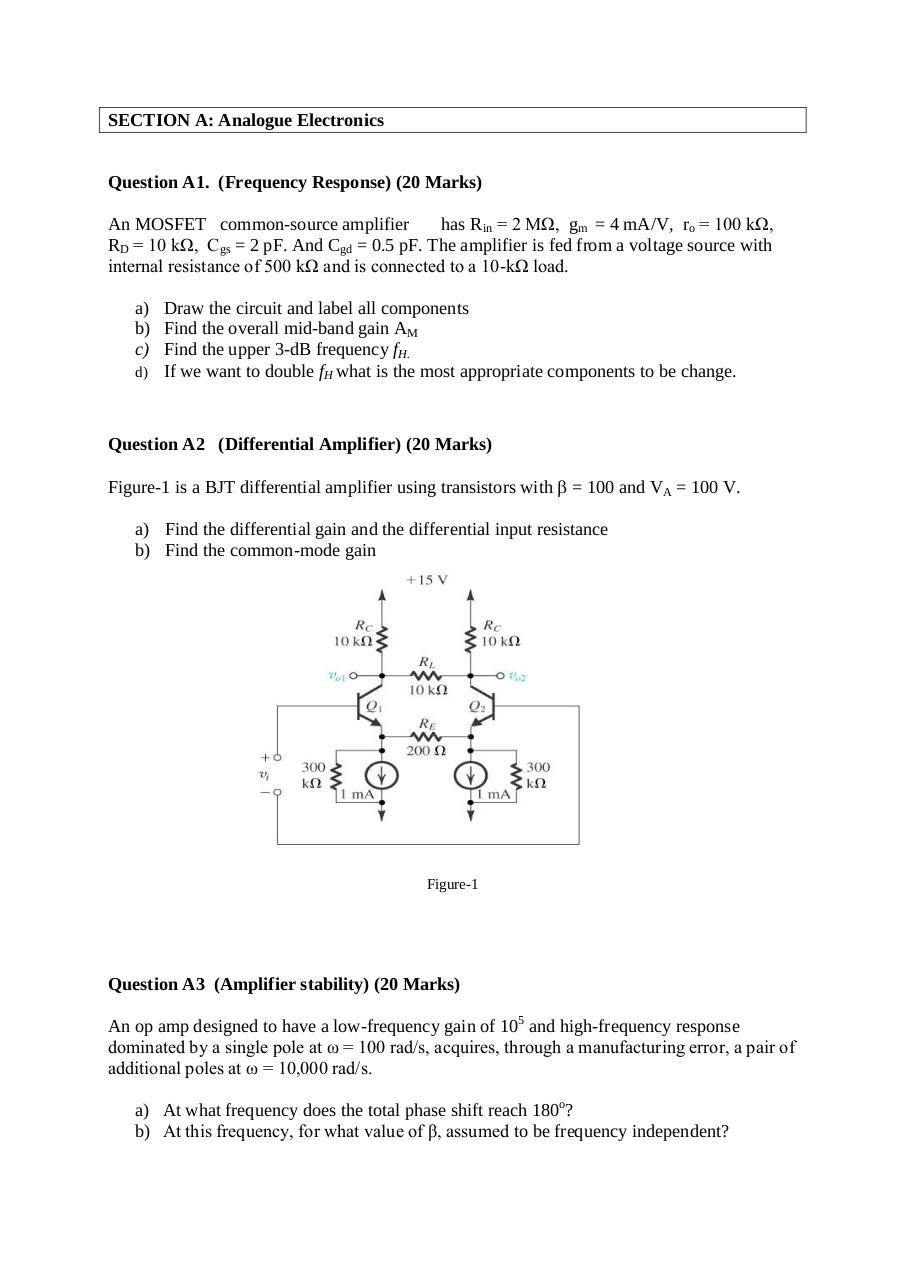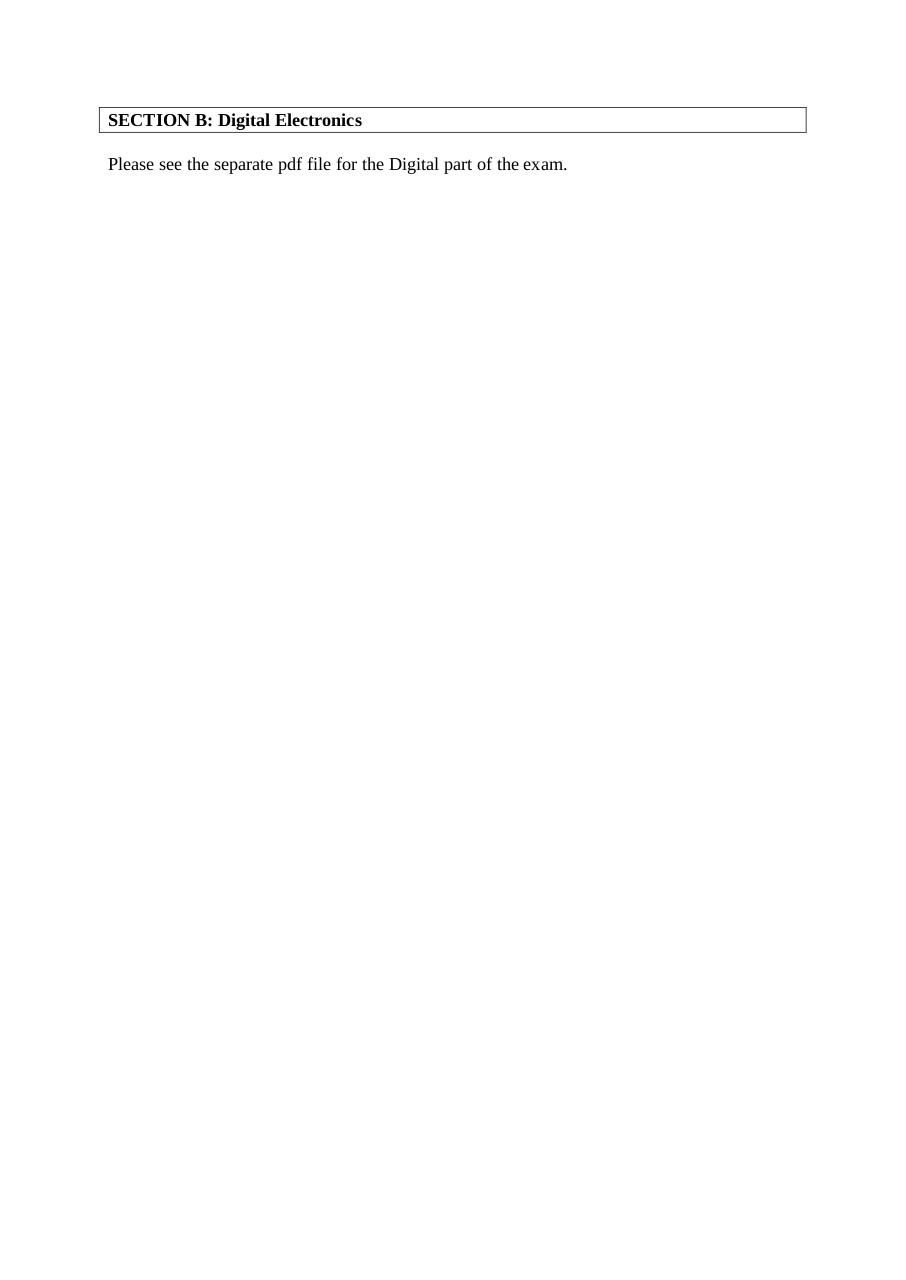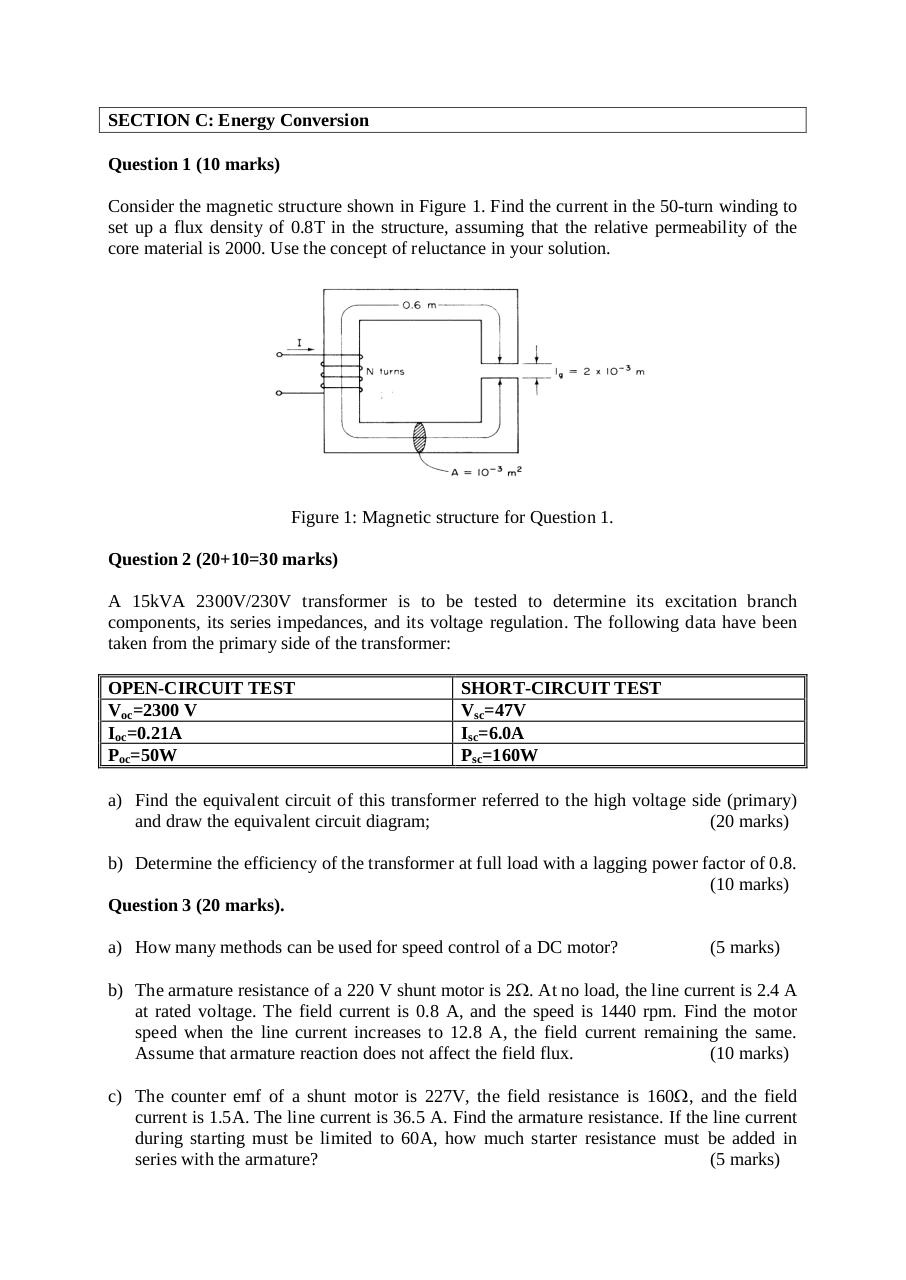#### HTML Code

Copy the following HTML code to share your document on a Website or Blog

#### QR Code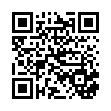### Related keywords